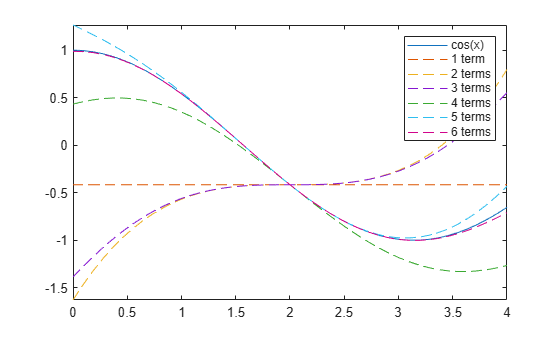# children

Subexpressions or terms of symbolic expression

Starting in R2020b, the syntax `subexpr = children(expr)` for a scalar input `expr` returns `subexpr` as a nonnested cell array instead of a vector. You can use ```subexpr = children(expr,ind)``` to index into the returned cell array of subexpressions. For more information, see Compatibility Considerations.

## Syntax

``subexpr = children(expr)``
``subexpr = children(A)``
``subexpr = children(___,ind)``

## Description

example

````subexpr = children(expr)` returns a nonnested cell array containing the child subexpressions of the symbolic expression `expr`. For example, the child subexpressions of a sum are its terms.```

example

````subexpr = children(A)` returns a nested cell array containing the child subexpressions of each expression in the symbolic matrix `A`.```

example

````subexpr = children(___,ind)` returns the child subexpressions of a symbolic expression `expr` or a symbolic matrix `A` as a cell array indexed by `ind`.```

## Examples

collapse all

Find the child subexpressions of the symbolic expression ${x}^{2}+xy+{y}^{2}$. The subexpressions are returned in a nonnested cell array. `children` uses internal sorting rules when returning the subexpressions. You can index into each element of the cell array by using `subexpr{i}`, where `i` is the cell index. The child subexpressions of a sum are its terms.

```syms x y subexpr = children(x^2 + x*y + y^2)```
```subexpr=1×3 cell array {[x*y]} {[x^2]} {[y^2]} ```
`s1 = subexpr{1}`
`s1 = $x y$`
`s2 = subexpr{2}`
`s2 = ${x}^{2}$`
`s3 = subexpr{3}`
`s3 = ${y}^{2}$`

You can also index into each element of the subexpressions by specifying the index `ind` in the `children` function.

`s1 = children(x^2 + x*y + y^2,1)`
`s1 = $x y$`
`s2 = children(x^2 + x*y + y^2,2)`
`s2 = ${x}^{2}$`
`s3 = children(x^2 + x*y + y^2,3)`
`s3 = ${y}^{2}$`

To convert the cell array of subexpressions into a vector, you can use the command `[subexpr{:}]`.

`V = [subexpr{:}]`
`V = $\left(\begin{array}{ccc}x y& {x}^{2}& {y}^{2}\end{array}\right)$`

Find the child subexpressions of the equation ${x}^{2}+xy={y}^{2}+1$. The child subexpressions of the equation are returned in a 1-by-2 cell array. Index into all elements of the cell array. The subexpressions of an equation are the left and right sides of that equation.

```syms x y subexpr = children(x^2 + x*y == y^2 + 1)```
```subexpr=1×2 cell array {[x^2 + y*x]} {[y^2 + 1]} ```
`subexpr{:}`
`ans = ${x}^{2}+y x$`
`ans = ${y}^{2}+1$`

Next, find the child subexpressions of the inequality $\mathrm{sin}\left(x\right)<\mathrm{cos}\left(x\right)$. Index into all elements of the returned cell array. The child subexpressions of an inequality are the left and right sides of that inequality.

`subexpr = children(sin(x) < cos(x))`
```subexpr=1×2 cell array {[sin(x)]} {[cos(x)]} ```
`subexpr{:}`
`ans = $\mathrm{sin}\left(x\right)$`
`ans = $\mathrm{cos}\left(x\right)$`

Find the child subexpressions of an integral ${\int }_{a}^{b}f\left(x\right)\phantom{\rule{0.2777777777777778em}{0ex}}dx$. The child subexpressions are returned as a cell array of symbolic expressions.

```syms f(x) a b subexpr = children(int(f(x),a,b))```
```subexpr=1×4 cell array {[f(x)]} {[x]} {[a]} {[b]} ```
`V = [subexpr{:}]`
`V = $\left(\begin{array}{cccc}f\left(x\right)& x& a& b\end{array}\right)$`

Find the Taylor approximation of the $\mathrm{cos}\left(x\right)$ function near $x=2$.

```syms x t = taylor(cos(x),x,2)```
```t =  $\mathrm{cos}\left(2\right)+\frac{\mathrm{sin}\left(2\right) {\left(x-2\right)}^{3}}{6}-\frac{\mathrm{sin}\left(2\right) {\left(x-2\right)}^{5}}{120}-\mathrm{sin}\left(2\right) \left(x-2\right)-\frac{\mathrm{cos}\left(2\right) {\left(x-2\right)}^{2}}{2}+\frac{\mathrm{cos}\left(2\right) {\left(x-2\right)}^{4}}{24}$```

The Taylor expansion has six terms that are separated by $+$ or $–$ sign.

Plot the $\mathrm{cos}\left(x\right)$ function. Use `children` to separate out the terms of the expansion. Show that the Taylor expansion approximates the function more closely as more terms are included.

```fplot(cos(x),[0 4]) hold on s = 0; for i = 1:6 s = s + children(t,i); fplot(s,[0 4],'--') end legend({'cos(x)','1 term','2 terms','3 terms','4 terms','5 terms','6 terms'})```Call the `children` function to find the child subexpressions of the following symbolic matrix input. The result is a `2`-by-`2` nested cell array containing the child subexpressions of each element of the matrix.

```syms x y symM = [x + y, sin(x)*cos(y); x^3 - y^3, exp(x*y^2) + 3]```
```symM =  $\left(\begin{array}{cc}x+y& \mathrm{cos}\left(y\right) \mathrm{sin}\left(x\right)\\ {x}^{3}-{y}^{3}& {\mathrm{e}}^{x {y}^{2}}+3\end{array}\right)$```
`s = children(symM)`
```s=2×2 cell array {1x2 cell} {1x2 cell} {1x2 cell} {1x2 cell} ```

To unnest or access the elements of the nested cell array `s`, use braces. For example, the `{1,1}-`element of `s` is a `1`-by-`2` cell array of symbolic expressions.

`s11 = s{1,1}`
```s11=1×2 cell array {[x]} {[y]} ```

Unnest each element of `s` using braces. Convert the nonnested cell arrays to vectors using square brackets.

`s11vec = [s{1,1}{:}]`
`s11vec = $\left(\begin{array}{cc}x& y\end{array}\right)$`
`s21vec = [s{2,1}{:}]`
`s21vec = $\left(\begin{array}{cc}{x}^{3}& -{y}^{3}\end{array}\right)$`
`s12vec = [s{1,2}{:}]`
`s12vec = $\left(\begin{array}{cc}\mathrm{cos}\left(y\right)& \mathrm{sin}\left(x\right)\end{array}\right)$`
`s22vec = [s{2,2}{:}]`
`s22vec = $\left(\begin{array}{cc}{\mathrm{e}}^{x {y}^{2}}& 3\end{array}\right)$`

If each element of the nested cell array `s` contains a nonnested cell array of the same size, then you can also use the `ind` input argument to access the elements of the nested cell array. The index `ind` allows `children` to access each column of subexpressions of the symbolic matrix input `symM`.

`scol1 = children(symM,1)`
```scol1=2×2 cell array {[x ]} {[cos(y) ]} {[x^3]} {[exp(x*y^2)]} ```
`[scol1{:}].'`
```ans =  $\left(\begin{array}{c}x\\ {x}^{3}\\ \mathrm{cos}\left(y\right)\\ {\mathrm{e}}^{x {y}^{2}}\end{array}\right)$```
`scol2 = children(symM,2)`
```scol2=2×2 cell array {[y ]} {[sin(x)]} {[-y^3]} {[3 ]} ```
`[scol2{:}].'`
```ans =  $\left(\begin{array}{c}y\\ -{y}^{3}\\ \mathrm{sin}\left(x\right)\\ 3\end{array}\right)$```

## Input Arguments

collapse all

Input expression, specified as a symbolic number, variable, function, or expression.

Input matrix, specified as a symbolic matrix.

Index of child subexpressions to return, specified as a positive integer.

• If `children(expr)` returns a nonnested cell array of child subexpressions, then indexing with `children(expr,ind)` returns the `ind`-th element of the cell array.

• If `children(A)` returns a nested cell array of child subexpressions, where each cell element has the same size, then indexing with `children(A,ind)` returns the `ind`-th column of the nonnested cell array.

## Version History

Introduced in R2012a

expand all